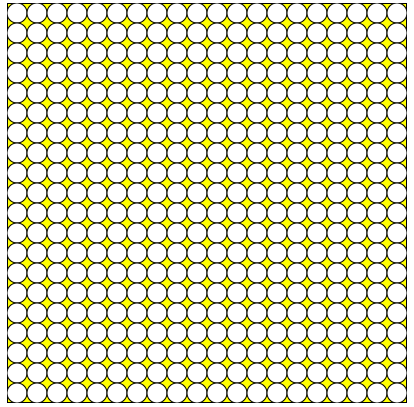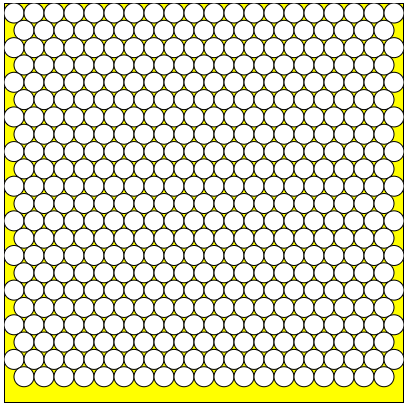Engineering ToolBox - Resources, Tools and Basic Information for Engineering and Design of Technical Applications!

# Circles within a Rectangle - Calculator

## Calculate the maximum number of circles within a rectangle - can be used to calculate the numbers of pipes or wires in a conduit or similar.

The calculator below estimates the maximum number of circles that may fit within a rectangle. The calculator can be used to calculate

• the number of pipes - or wires - that fits within a conduit or similar applications

Input the rectangle inside dimensions - height and width and the circles outside diameters.

Default values are for 0.5 inch circles inside a 10 inch x 10 inch square. The calculator is generic and any kind of units can be used - as long as the same units are used for all values.

w - rectangle width (in, mm, m)

h - rectangle height (in, mm, m)

d - circle diameter (in, mm, m)

s - space between circles - and between circles and rectangle walls (in, mm, m)

### Rectangular Pattern#### Rectangular Pattern - Circle Coordinates

Copy and paste the circle center coordinates to your application. x = 0 and y = 0 is top left corner of rectangle.

x y

Tip! - the values can be adapted and modified in excel or in a text editor for use in a CNC G-code generator or similar.

### Triangular Pattern#### Triangular Pattern - Circle Coordinates

Copy and paste the circle center coordinates to your application. x = 0 and y = 0 is top left corner of rectangle.

x y

Be aware! - The algorithm is quite simple - switching rectangle width and height may influence the number calculated. Switching the input values above changes the layout and gives

Note! - with some combinations of rectangular shapes and circle sizes - one or two more circles - or even more - may be added with a modified layout of the circles. In the default triangular example above - two more circles can be added in between if the left and right bottom circles are moved to the left and right border. The algorithm used for the calculation is quite simple and may underestimate the number of circles in some cases.

## Related Topics

• Mathematics - Mathematical rules and laws - numbers, areas, volumes, exponents, trigonometric functions and more.
• Electrical - Electrical units, amps and electrical wiring, wire gauge and AWG, electrical formulas and motors.

## Engineering ToolBox - SketchUp Extension - Online 3D modeling!

Add standard and customized parametric components - like flange beams, lumbers, piping, stairs and more - to your Sketchup model with the Engineering ToolBox - SketchUp Extension - enabled for use with the amazing, fun and free SketchUp Make and SketchUp Pro .Add the Engineering ToolBox extension to your SketchUp from the SketchUp Pro Sketchup Extension Warehouse!

Translate

## Privacy

We don't collect information from our users. Only emails and answers are saved in our archive. Cookies are only used in the browser to improve user experience.

Some of our calculators and applications let you save application data to your local computer. These applications will - due to browser restrictions - send data between your browser and our server. We don't save this data.

## Citation

• Engineering ToolBox, (2014). Circles within a Rectangle - Calculator. [online] Available at: https://www.engineeringtoolbox.com/circles-within-rectangle-d_1905.html [Accessed Day Mo. Year].

Modify access date.

. .

#### Scientific Online Calculator3 30